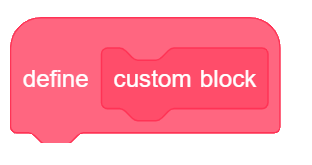My Blocks allows you to define a sequence of actions which are performed when called. In programming this is called a ‘procedure’.

Adaptable procedures extend ‘My Blocks’ by passing values to the procedures, which are used as variables. In the code here, the ‘Shape 4 60’ block calls the Shape procedure (set of instructions) passing two values to it. These are then used within the block to perform the instructions.

You need to …

• Understand Loops and some Pen extension of Scratch
• Be taught about defining My Blocks using number variables
• Have a basic understanding of x-y coordinates in more than one quadrant.

This unit introduces the following code blocks

## Results

### Investigate Further

Make sure you know what each part does

### Modify the Code

Change the shapes to create a triangle, heptagon or decagon Make it draw four pentagons of different sizes

###Look closely at the code and make sure you understand how it works.

### Investigate Further

Make sure you know what each part does

### Modify the Code

Change the shapes to create a triangle, heptagon or decagon

Make it draw four pentagons of different sizes

To modify the `code,` click here

### #1. What shape do you think this will draw? ? The 'Shape 4 60' command on the right is passed to the Shape procedure (algorithm)

‘Shape 4 60’ will tell the Shape procedure (My BLock) to draw a Shape with 4 sides and length of 60.

The angle will be ‘360 ÷ sides’ or 360 ÷ 4 which is 90 degrees ( a right angle)

The sides will all be ‘move length steps’ and, as the length is 60, they will move 60 steps.

Hence it will draw a square.

### #2. How much will the sprite turn right each time it is called in this code? ? Look at 'TurnThis command creates the angle of turn in the shape.
It will turn 360 ÷ sides

As sides=6, it will turn 360° ÷ 6 = 60°

Please note this is the exterior angle that it will turn.

### #3. How many steps will it move each time? ? Look at the 'move length steps' blockThis is the instruction which draws each side and tells it how long it should be.This instruction tells it to draw a Shape with 8 sides, each of length 30.

So each time it runs the ‘Steps’ command block, it will ‘move 30 steps’

### #4. Which block was used with the function to create this shape? ? What shape is it? That is a big clue.

The shape is an Octogon, which has 8 sides.

The first number passed to the My Block procedure defines how many repeats it will do, and therefore how many sides it will draw.With this command, the number of sides is 8, so it will repeat 8 times drawing sides 30 steps in length.

### #5. This code will put different shapes in different places, what will it look like? ? What do you know about coordinates? Think positive!These commands tell the Sprite where to go before drawing…

• If the numbers are both positive, it will draw in the top right.
• If the x value is negative, it will draw on the left.
• If the y value is negative, it will draw at the bottom

When both x and y are negative, it moves to the bottom left of the screen and draws a shape with 6 sides – a hexagon.This page’s content is closely aligned to the Code-IT resources designed by Phil Bagge.
http://code-it.co.uk/goldshape/#BasicProceedures